## Filters

Sort by :
Clear All
Q

A triangular prism looks like the shape of a Kaleidoscope. It has triangles as its bases.

Faces : _______

Edges : _______

Corners : _______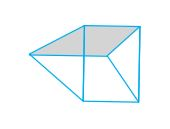Faces =   (Two triangular faces and three square faces) Edges = 9     Corners =

A square pyramid has a square as its base.

Faces : _______

Edges : _______

Corners : _______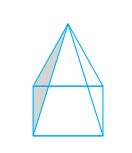In a square pyramid, the number of Faces =           (Four triangular faces and one square face) Edges =           (Four edges of the square base and other four joining at the top)    Corners =

A triangular pyramid has a triangle as its base. It is also known as a tetrahedron.

Faces : _______

Edges : _______

Corners : _______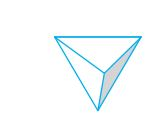The number of Faces =           (All triangular faces) Edges =               Corners =

A cube is a cuboid whose edges are all of equal length.

It has ______ faces.

Each face has ______ edges.

Each face has ______ vertices.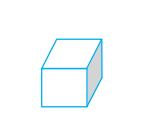It has  faces.        (Three pairs of parallel square faces)   Each face has  edges.          Each face has  vertices

Using four unequal sticks, as you did in the above activity, see if you can form a quadrilateral such that

(a) all the four angles are acute.

(b) one of the angles is obtuse.

(c) one of the angles is right angled.

(d) two of the angles are obtuse.

(e) two of the angles are right angled.

(f) the diagonals are perpendicular to one another

(a) all the four angles are acute.             (b) one of the angles is obtuse.             (c) one of the angles is right angled.             (d) two of the angles are obtuse.               (e) two of the angles are right angled.               (f) the diagonals are perpendicular to one another

Place a pair of unequal sticks such that they have their end points joined at one end. Now place another such pair meeting the             free ends of the first pair. What is the figure enclosed? It is a quadrilateral, like the one you see here. The sides of the                      quadrilateral are AB, BC , ___, ___. There are 4 angles for this quadrilateral. They are given by ∠BAD, ∠ADC, ∠DCB and _____.            BD is one diagonal. What is the other? Measure the length of the sides and the diagonals. Measure all the angles also.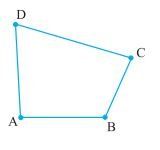The sides of the quadrilateral are AB, BC, CD, DA The angles are given by The other diagonal is AD.

Try to draw rough sketches of

(a) a scalene acute angled triangle.

(b) an obtuse angled isosceles triangle.

(a) a scalene acute angled triangle. :  Scalene : All side of different length Acute angled : All angles less than    (b) an obtuse angled isosceles triangle Isosceles traingle: Only two sides are of equal length Obtuse angled : At least one angle greater than

What does the angle made by the hour hand of the clock look like when it moves from 5 to 7. Is the angle moved more than 1                   right angle?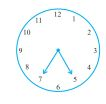No, the angle is not more than than 1 right angle.  For each hour, angle made =  Therefore, when the hour hand moves from 5 to 7, the angle made =

The hour hand of a clock moves from 12 to 5. Is the revolution of the hour hand more than 1 right angle?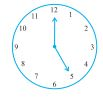Yes, the revolution of the hour hand is more than 1 right angle. For each hour, angle made =  Therefore, when the hour hand moves from 12 to 5, the angle made =

Draw five other situations of one-fourth, half and three-fourth revolution on a clock.

(a) One fourth revolution: From (b) Half revolution: From (c) Three fourth revolution: From (d) Three fourth revolution: From (e) Half fourth revolution: From

What is the angle name for one-fourth revolution?

One-fourth revolution =  The angle name for one-fourth revolution is "Right Angle"

What is the angle name for half a revolution?

Half a revolution =  The angle name for half a revolution is "Straight Angle".

1.   Match the following :

(a) Cone                                                          (i)(b) Sphere                                                      (ii)(c) Cylinder                                                     (iii)(d) Cuboid                                                       (iv)(e) Pyramid                                                      (v)(a) Cone                                                  (ii)           (b) Sphere                                               (iv)          (c) Cylinder                                              (v)        (d) Cuboid                                             (iii)        (e) Pyramid                                            (i)

5.  A diagonal is a line segment that joins any two vertices of the polygon and is not a side of the polygon. Draw a rough sketch of a pentagon and draw its diagonals.

We have drawn the pentagon ABCDE and by joining its vertices he has drawn the diagonals AC, CE, EB, BD and DA.

4. Draw a rough sketch of a regular octagon. (Use squared paper if you wish). Draw a rectangle by joining exactly four of the vertices of the octagon.

We have made the regular octagon ABCDEFGH and by joining vertices H, C, D and G we have formed the rectangle HCDG

3. Draw a rough sketch of a regular hexagon. Connecting any three of its vertices, draw a triangle. Identify the type of the triangle you have drawn.

We have drawn the regular Hexagon ABCDEF and by joining the vertices B, D and F we have formed the Equilateral Triangle BDF.

2.  Name each polygon.Make two more examples of each of these.

(a) Quadrilateral (b) Triangle (c) Pentagon (d) Octagon

1.   Examine whether the following are polygons. If any one among them is not, say why?(a) The given figure is not a polygon as it is not a closed figure. (b) The given figure is  a polygon. (c) The given figure is not a polygon as a polygon is enclosed only by line segments. (d) The given figure is not a polygon as a polygon is enclosed only by line segments.

3.   A figure is said to be regular if its sides are equal in length and angles are equal in measure. Can you identify the regular quadrilateral?

Square is the only quadrilateral with sides equal in length and angles equal in measure, therefore, a square is the regular quadrilateral.

2.(e) Give reasons for the following :

(e)     Square is also a parallelogram.

Square is also a parallelogram as its opposite sides are parallel.
Exams
Articles
Questions